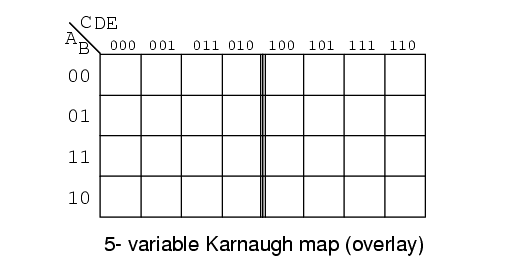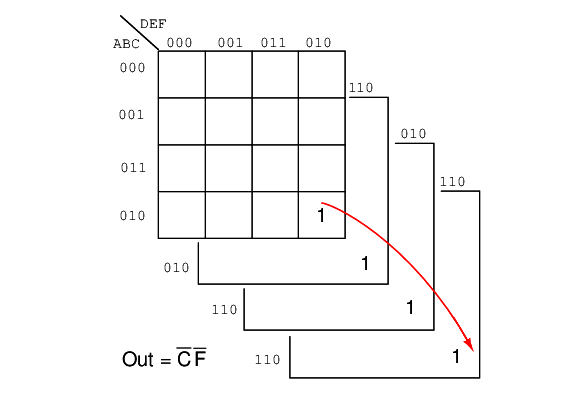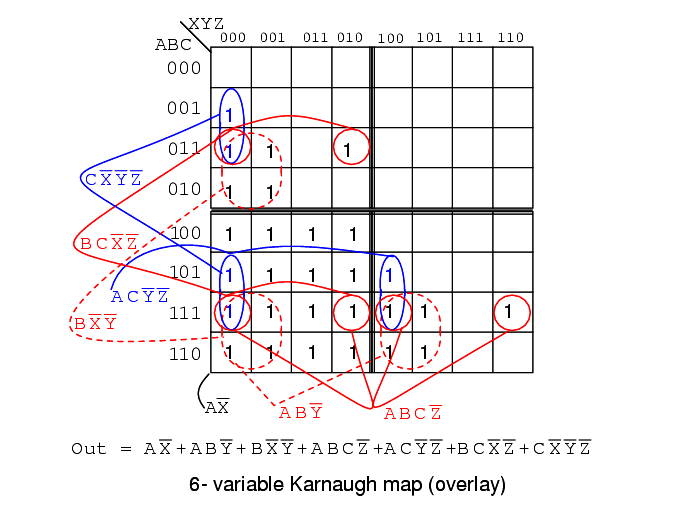# Larger 5 & 6-variable Karnaugh Maps

You are here:

Larger Karnaugh maps reduce larger logic designs. How large is large enough? That depends on the number of inputs, fan-ins, to the logic circuit under consideration. One of the large programmable logic companies has an answer.

Altera’s own data, extracted from its library of customer designs, supports the value of heterogeneity. By examining logic cones, mapping them onto LUT-based nodes and sorting them by the number of inputs that would be best at each node, Altera found that the distribution of fan-ins was nearly flat between two and six inputs, with a nice peak at five.

## 5 Variable Karnaugh Map

The answer is no more than six inputs for most all designs, and five inputs for the average logic design. The five variable Karnaugh map follows.The older version of the five variable K-map, a Gray Code map or reflection map, is shown above. The top (and side for a 6-variable map) of the map is numbered in full Gray code.

The Gray code reflects about the middle of the code. This style map is found in older texts.

The newer preferred style is below.The overlay version of the Karnaugh map, shown above, is simply two (four for a 6-variable map) identical maps except for the most significant bit of the 3-bit address across the top. If we look at the top of the map, we will see that the numbering is different from the previous Gray code map.

If we ignore the most significant digit of the 3-digit numbers, the sequence 00, 01, 11, 10 is at the heading of both sub maps of the overlay map. The sequence of eight 3-digit numbers is not Gray code. Though the sequence of four of the least significant two bits is.

Let’s put our 5-variable Karnaugh Map to use. Design a circuit which has a 5-bit binary input (A, B, C, D, E), with A being the MSB (Most Significant Bit). It must produce an output logic High for any prime number detected in the input data.We show the solution above on the older Gray code (reflection) map for reference. The prime numbers are (1,2,3,5,7,11,13,17,19,23,29,31). Plot a 1 in each corresponding cell. Then, proceed with grouping of the cells. Finish by writing the simplified result.

Note that 4-cell group A’B’E consists of two pairs of cell on both sides of the mirror line. The same is true of the 2-cell group AB’DE. It is a group of 2-cells by being reflected about the mirror line. When using this version of the K-map look for mirror images in the other half of the map.

Out = A’B’E + B’C’E + A’C’DE + A’CD’E + ABCE + AB’DE + A’B’C’D

Below we show the more common version of the 5-variable map, the overlay map.If we compare the patterns in the two maps, some of the cells in the right half of the map are moved around since the addressing across the top of the map is different. We also need to take a different approach at spotting commonality between the two halves of the map.

Overlay one half of the map atop the other half. Any overlap from the top map to the lower map is a potential group. The figure below shows that group AB’DE is composed of two stacked cells. Group A’B’E consists of two stacked pairs of cells.

For the A’B’E group of 4-cells ABCDE = 00xx1 for the group. That is A,B,E are the same 001 respectively for the group. And, CD=xx that is it varies, no commonality in CD=xx for the group of 4-cells. Since ABCDE = 00xx1, the group of 4-cells is covered by A’B’XXE = A’B’E.The above 5-variable overlay map is shown stacked.

## 6 variable Karnaugh map

An example of a six variable Karnaugh map follows. We have mentally stacked the four sub maps to see the group of 4-cells corresponding to Out = C’F’A magnitude comparator (used to illustrate a 6-variable K-map) compares two binary numbers, indicating if they are equal, greater than, or less than each other on three respective outputs.

A three bit magnitude comparator has two inputs A2A1A0 and B2B1B0 An integrated circuit magnitude comparator (7485) would actually have four inputs, But, the Karnaugh map below needs to be kept to a reasonable size. We will only solve for the A>B output.

Below, a 6-variable Karnaugh map aids simplification of the logic for a 3-bit magnitude comparator. This is an overlay type of map. The binary address code across the top and down the left side of the map is not a full 3-bit Gray code.

Though the 2-bit address codes of the four sub maps is Gray code. Find redundant expressions by stacking the four sub maps atop one another (shown above). There could be cells common to all four maps, though not in the example below. It does have cells common to pairs of sub maps.The A>B output above is ABC>XYZ on the map below.Where ever ABC is greater than XYZ, a 1 is plotted. In the first line ABC=000 cannot be greater than any of the values of XYZ. No 1s in this line. In the second line, ABC=001, only the first cell ABCXYZ= 001000 is ABC greater than XYZ. A single 1 is entered in the first cell of the second line.

The fourth line, ABC=010, has a pair of 1s. The third line, ABC=011 has three 1s. Thus, the map is filled with 1s in any cells where ABC is greater than XXZ.

In grouping cells, form groups with adjacent sub maps if possible. All but one group of 16-cells involves cells from pairs of the sub maps. Look for the following groups:

• 1 group of 16-cells
• 2 groups of 8-cells
• 4 groups of 4-cells

The group of 16-cells, AX’ occupies all of the lower right sub map; though, we don’t circle it on the figure above.

One group of 8-cells is composed of a group of 4-cells in the upper sub map overlaying a similar group in the lower left map. The second group of 8-cells is composed of a similar group of 4-cells in the right sub map overlaying the same group of 4-cells in the lower left map.

The four groups of 4-cells are shown on the Karnaugh map above with the associated product terms. Along with the product terms for the two groups of 8-cells and the group of 16-cells, the final Sum-Of-Products reduction is shown, all seven terms.

Counting the 1s in the map, there is a total of 16+6+6=28 ones. Before the K-map logic reduction there would have been 28 product terms in our SOP output, each with 6-inputs. The Karnaugh map yielded seven product terms of four or less inputs. This is really what Karnaugh maps are all about!

The wiring diagram is not shown. However, here is the parts list for the 3-bit magnitude comparator for ABC>XYZ using 4 TTL logic family parts:

• 1 ea 7410 triple 3-input NAND gate AX’, ABY’, BX’Y’
• 2 ea 7420 dual 4-input NAND gate ABCZ’, ACY’Z’, BCX’Z’, CX’Y’Z’
• 1 ea 7430 8-input NAND gate for output of 7-P-terms

#### Summary

Boolean algebra, Karnaugh maps, and CAD (Computer Aided Design) are methods of logic simplification. The goal of logic simplification is a minimal cost solution.

A minimal cost solution is a valid logic reduction with the minimum number of gates with the minimum number of inputs.

Venn diagrams allow us to visualize Boolean expressions, easing the transition to Karnaugh maps.

Karnaugh map cells are organized in Gray code order so that we may visualize redundancy in Boolean expressions which results in simplification.

The more common Sum-Of-Products (Sum of Minters) expressions are implemented as AND gates (products) feeding a single OR gate (sum).

Sum-Of-Products expressions (AND-OR logic) are equivalent to a NAND-NAND implementation. All AND gates and OR gates are replaced by NAND gates.

Less often used, Product-Of-Sums expressions are implemented as OR gates (sums) feeding into a single AND gate (product). Product-Of-Sums expressions are based on the 0s, maxterms, in a Karnaugh map.